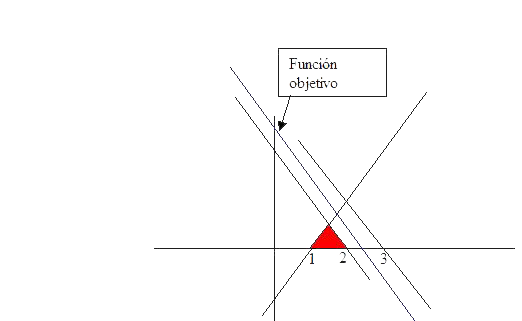Linear Programming Samples

Infinite solutions Sample

Lets consider
Maximize (x + y)
Subject to
x + y ≤ 3
x + y ≤ 2
x - y ≥ 1
x, y ≥ 0

The Feasible regionThe feasible region is bounded and nonempty.
As shown in the graph, the objective function is parallel to the lines that limit the feasible region and grows in the direction of growth of x and y coordinates the maximum is reached in any one of the points of the feasible set located at the line farthest from the origin of coordinates point, thus for all these points the objective function takes the same value, therefore have an entire segment of optimal solutions, ie we are at infinite solution case.

Was useful? want add anything?

Post here

Post from other users

Post here